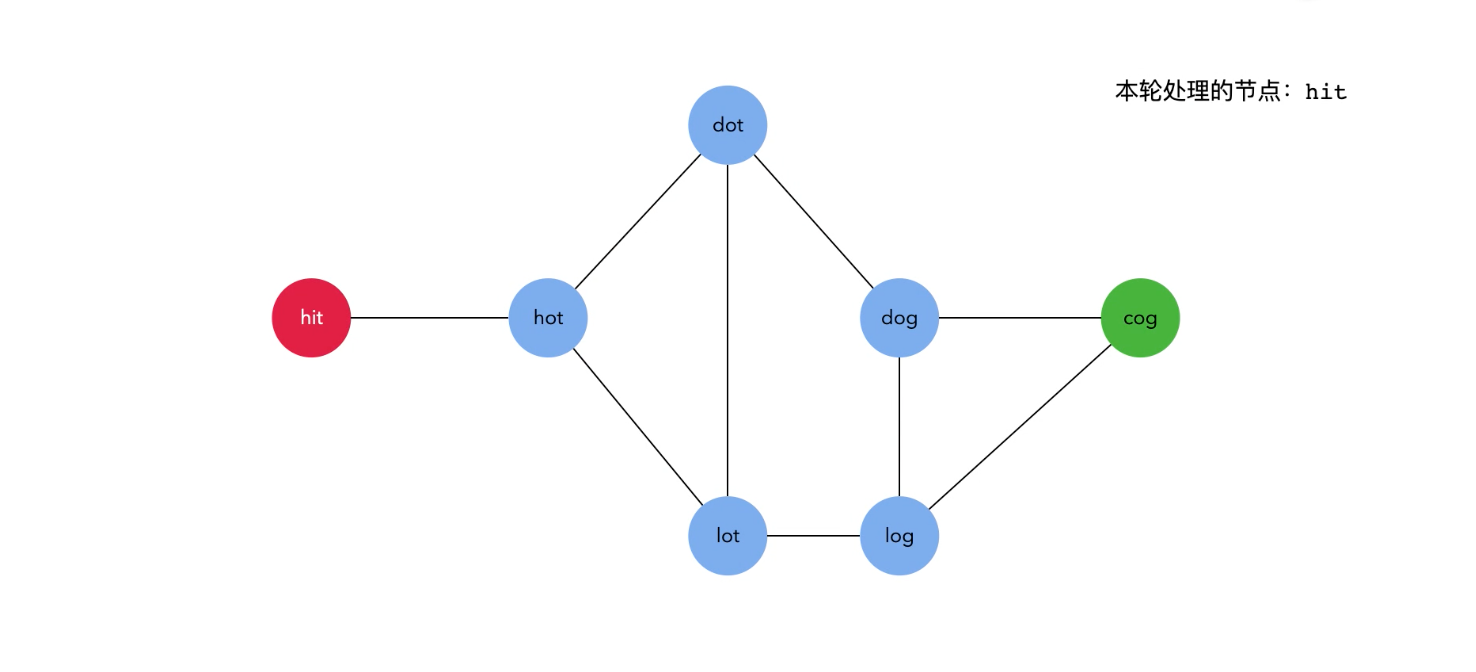# 搞定算法与数据结构（03）：BFS（广度优先查找）最短路径案例模板

## 给定问题

• 序列中第一个单词是 beginWord 。
• 序列中最后一个单词是 endWord 。
• 每次转换只能改变一个字母。
• 转换过程中的中间单词必须是字典 wordList 中的单词。

输入：beginWord = "hit", endWord = "cog", wordList = ["hot","dot","dog","lot","log","cog"]



输入：beginWord = "hit", endWord = "cog", wordList = ["hot","dot","dog","lot","log"]



## 解题思路

wordList = ["hot","dot","dog","lot","log"]wordList = ["hot","dot","dog","lot","log","cog"]

def construct_dict():
map = {}
for word in wordList:
for index in range(len(word)):
s = word[:index] + "_" + word[index+1:]
map[s] = map.get(s, []) + [word]
return map

construct_dict()


{
'_og': ['dog', 'log', 'cog'],
'_ot': ['hot', 'dot', 'lot'],
'c_g': ['cog'],
'co_': ['cog'],
'd_g': ['dog'],
'd_t': ['dot'],
'do_': ['dot', 'dog'],
'h_t': ['hot'],
'ho_': ['hot'],
'l_g': ['log'],
'l_t': ['lot'],
'lo_': ['lot', 'log']
}


def bfs(begin, target, d):
queue, visited = [(begin, 1)], set()
while len(queue) > 0:
word, steps = queue.pop(0)
if word not in visited:
if word == target:
return steps
for i in xrange(len(word)):
s = word[:i] + "_" + word[i+1:]
for j in d.get(s, []):
# 如果j在visited中，说明j已经被访问过了，不需要再次进行访问。
if j not in visited:
queue.append((j, steps+1))
return 0


## 经验总结

• 已访问元素的集合，以便判断某些元素已经访问过了，重复访问永远不可能是最短路径
• 待访问元素的集合，以便一轮访问完成后，知道下一轮要访问哪些元素
• 能够直接或间接表示图结构的数据结构，如上面的 map